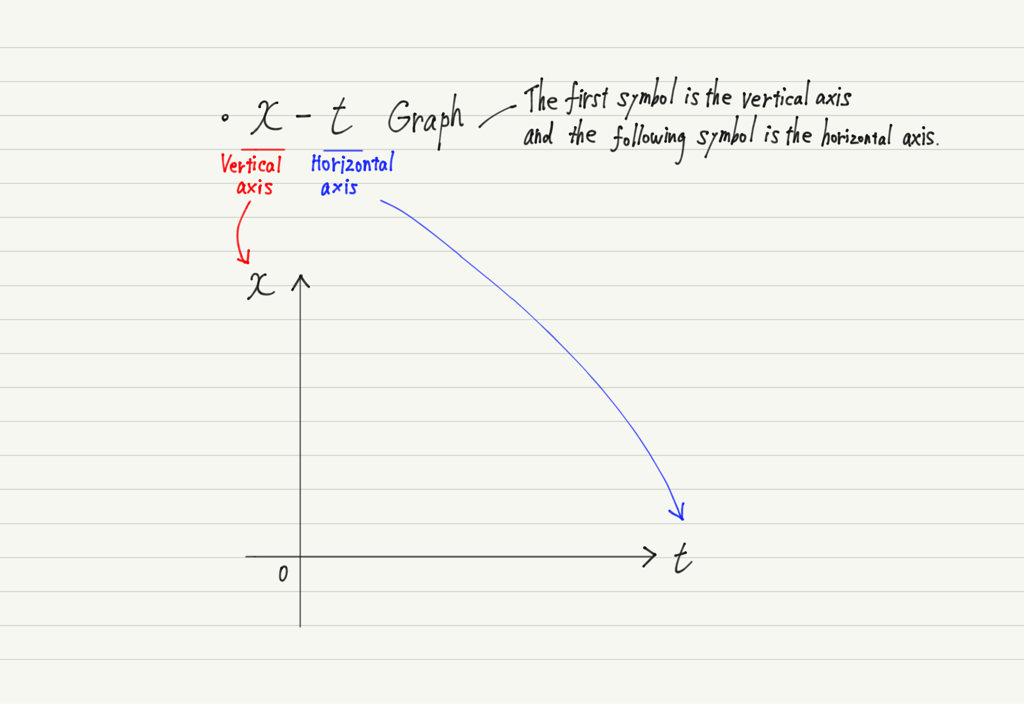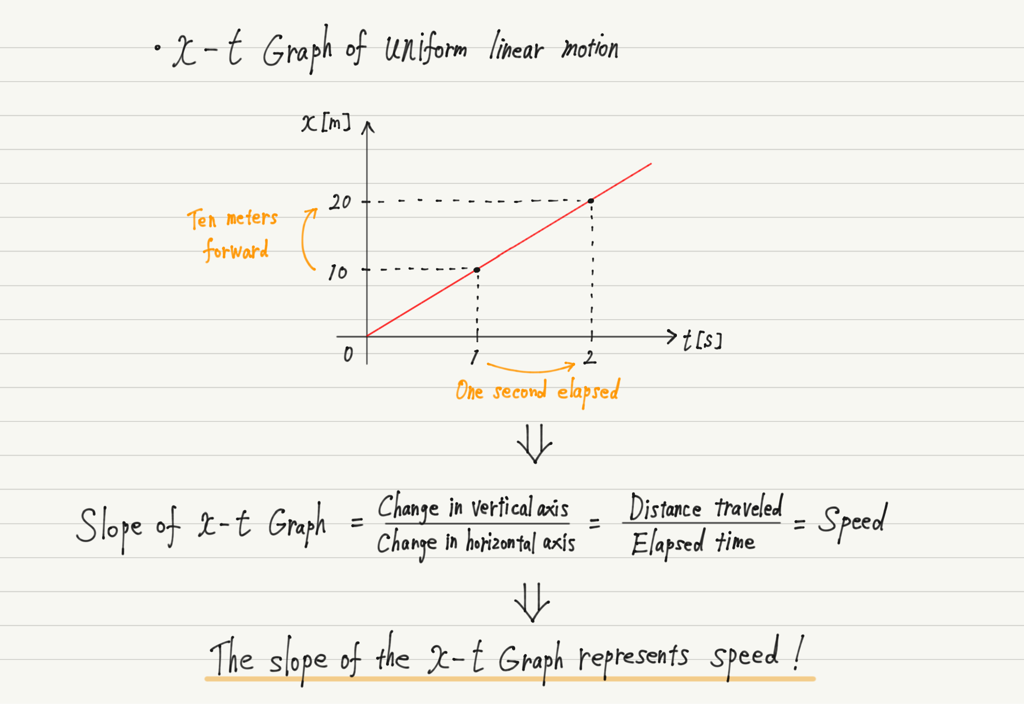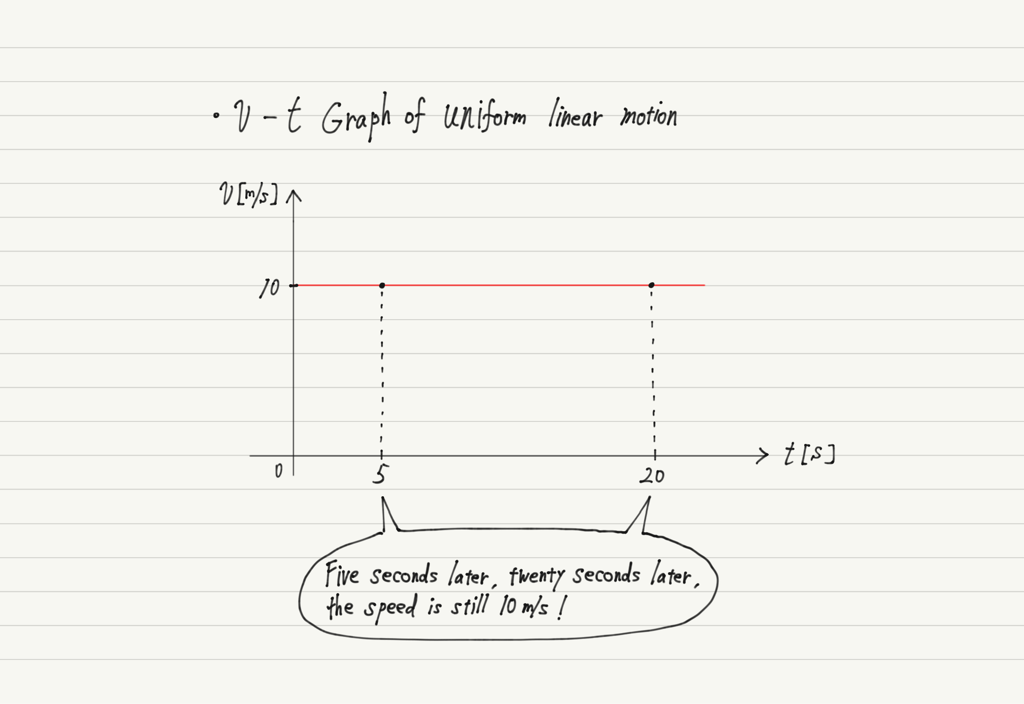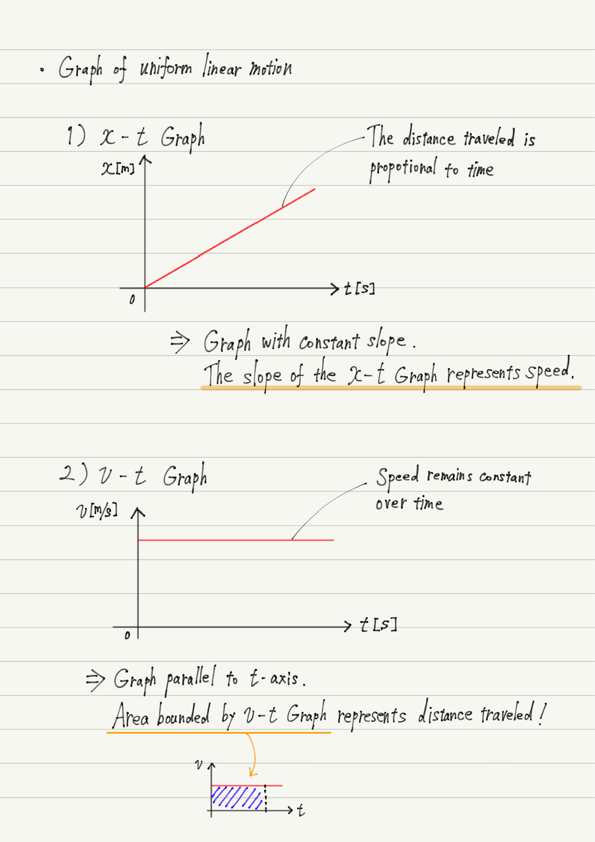# Graph of Uniform Linear Motion

(This article was translated from Japanese to English using DeepL.)

In this lecture, we will consider graphing uniform linear motion.

Some people are intimidated when they hear the word “graph,” but it is not difficult at all.
Using graphs, you can visualize motion, solve problems that would be difficult to calculate directly, and more!

## ① x-t Graph

The three main components of uniform linear motion are speed, distance, and time, but since we cannot graph all three together, we will pick up two of the three and graph them.
So, first of all, here is a graph showing the relationship between x(Distance) and t(Time).What will be the shape of the graph?

For example, if we consider an object moving at a constant speed of 10 m/s, this means that it will move 10 m per second, so it will move 20 m in 2 seconds, 30 m in 3 seconds, … , 100 m in 10 seconds.
Thus, as time doubles or triples, the distance traveled also doubles or triples. That shows proportionality!

In uniform linear motion, the distance traveled increases in proportion to the time taken, so the x-t graph becomes a graph of a straight line.It is important to note that the slope of the x-t graph represents speed. The greater the slope of the graph, the faster the motion.

## ② v-t Graph

Next is a graph showing the relationship between v (Speed) and t (Time).

This is a graph showing “How does the velocity change with time?”
Since this is a uniform linear motion, the speed does not change no matter how much time passes.

Considering an object moving at a constant speed of 10 m/s as before, the graph is as follows.It’s a very simple graph.

Now, let’s separate this graph at an appropriate time (e.g., t=20 s).Thus, the area enclosed by the v-t graph and the t-axis represents the distance traveled!

This will be used later, so please keep it in mind. That’s all for this lecture!

## Summary of this lecture## Next Time

Next time I would like to stop and review some of the terms.

https://www.yukimura-physics.com/entry/dyn-f03

error: Content is protected !!